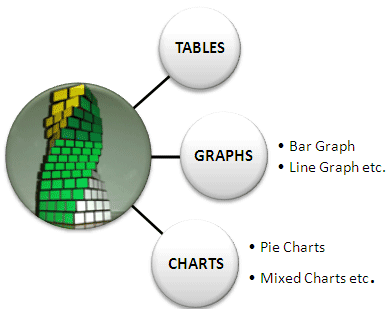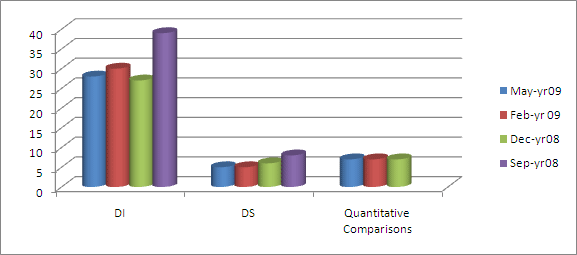# Data Interpretation & Data Sufficiency:MAT Exam
Explore more-MAT
KI Unique
Test Yourself
Help

## DATA INTERPRETATION & DATA SUFFICIENCY

This section not only contains questions on DS or DI, but questions on Quantitative Comparisons also become part of it. This section contains 40 questions, which are to be answered in 35 minutes.

### DATA SUFFICIENCY:

Data Sufficiency tests the quantitative reasoning proficiency using some unusual set of instructions. The examinee is provided with a question with 2 associated statements that may help in answering the question. It is on the examinee to decide that whether the 1st statement alone is sufficient to answer or the 2nd statement alone is sufficient or both are required in parts and so on.

### ANSWER TO DATA SUFFICIENCY QUESTIONS

1. Choice 1 if the question can be answered using one of the statements alone, while the other statement is not sufficient to answer the question.
2. Choice 2 if the question can be answered using each of the statements independently.
3. Choice 3 if both the statements together are needed to answer the question.
4. Choice 4 if both the statements independently or taken together are not sufficient to answer the question.

This type of question tests confirms candidate’s ability to understand and ability to identify the missing data of problem. DS problems are generally tricky.

### DATA INTERPRETATION: DI

• This is the calculation intensive portion of the section.
• The key to cracking this area is to quickly identify the key pieces of data that you will require to work on the questions asked.
• In this section data is presented before you in the various forms such as tables, Pie charts, graphs.etc.
• A candidate has to interpret the data and answer the question followed by data.
• This section mainly tests candidates the ability to interpret and act according to given data.

### The DATA can be presented in any of the forms mentioned below.Mostly, not all questions in a set are of equal difficulty.

### QUANTITATIVE COMPARISONS:

Here the examinee is not required to solve a problem for a particular value.

• As the name suggests, here the examinee has to make comparisons among two quantities.
• Each quantitative comparison displays two mathematical expressions in boxes—one in Column A and one in Column B.
• Sometimes additional information is also provided.

### MAT DI & DS ANALYSIS:Copy Right © 2012 by Knowledge Icon
Website Designed & Developed By Dream Business Systems